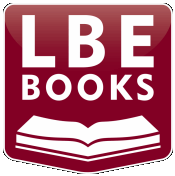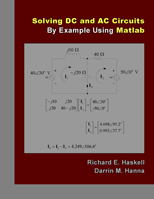The fast way to master modern engineering toolsBOOKS & KITS
DC and AC Circuits / Matlab

There is 1 book in this category

click the 'Add to Cart' button to purchase a book
click the 'View Cart' button to view your cart or proceed to checkout
use the toolbar on the left to change categories

 Solving DC and AC Circuits By Example Using Matlab Richard E. Haskell and Darrin M. Hanna (246 pages) This book uses Matlab as an aid to learning and understanding basic circuit analysis in over 50 Examples solving DC and AC Circuits.  We start by introducing Ohm’s law and Kirchhoff’s law while putting these laws and other basic concepts in a historical perspective.  This will provide you with the knowledge of where the basic ideas of electrical science come from. Following are circuit elements including resistors, capacitors, and inductors.  Sinusoidal signals and phasors are introduced and the concept of RMS values are described.  This allows both DC and steady-state AC circuits to be solved in subsequent chapters.  Examples involving voltage dividers, current division, and source transformation are given.  Circuits that include an ideal operational amplifier (op amp) are described.  Mesh analysis, nodal analysis, superposition, Thevenin's theorem, Norton's theorem, and maximum power transfer are covered.  Average power and transformers are covered along with the theory behind the operation of DC motors.  Basic material on vectors and matrices are included in the Appendices.  By restricting the analysis of AC circuits to sinusoidal signals we do not require any knowledge of differential equations and we leave transient analysis to a later course.  All circuit problems in this book contain only independent voltage and current sources.   All worked examples in the book show the calculations using Matlab. The use of Matlab clarifies all of the steps.  We also use Matlab to create a variety of plots that will help you understand the material.Preface Table of Contents Only \$39.95 Special Introductory offer \$29.95

By ordering any of our books, you will receive reminders and discounts on book packets containing updated tutorials for new releases of software, prototyping boards, and other tools.